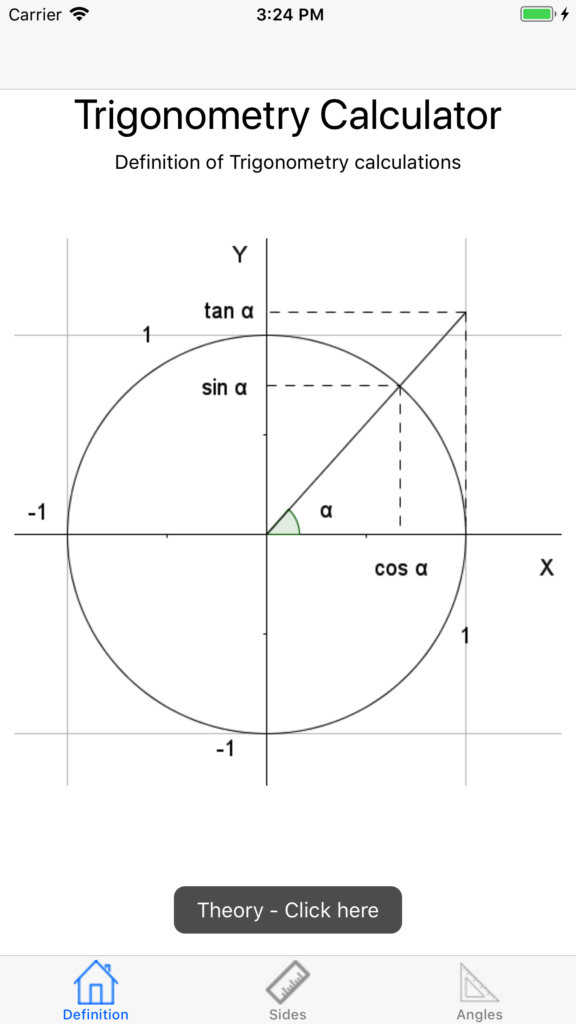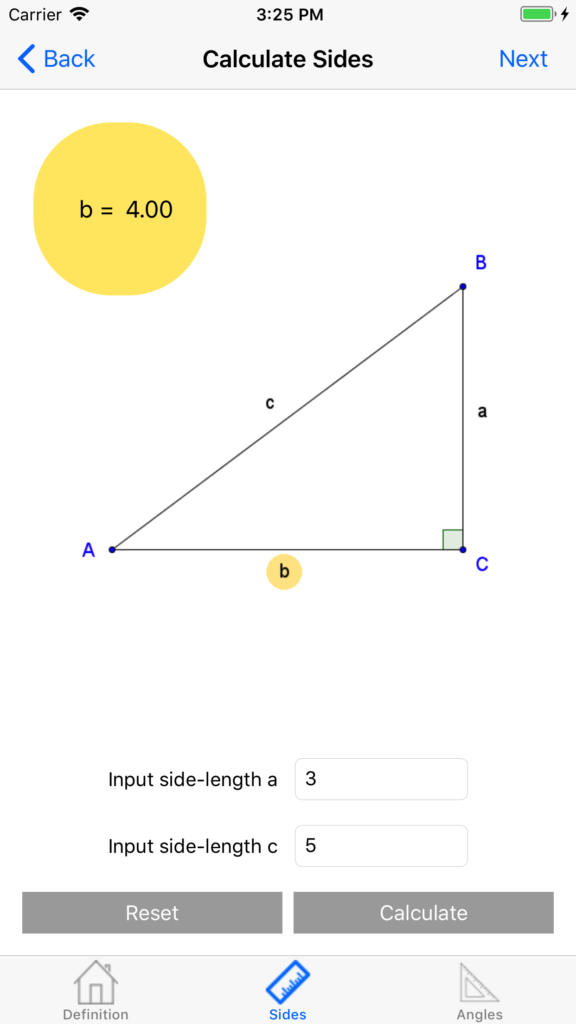# Trigonometry Calc

Use Trigonometry Calc to calculate sidelengths and angles in the right triangle.

••••Use Trigonometry Calc to calculate sidelengths and angles in the right triangle.

By the help of Pythagorean Theorem and some trigonometry functions you can in this app calculate side lengths and angles in any right triangle you like.

Learn some basic trigonometry.

•Math
•Trigonometry
•Pythagorean Theorem